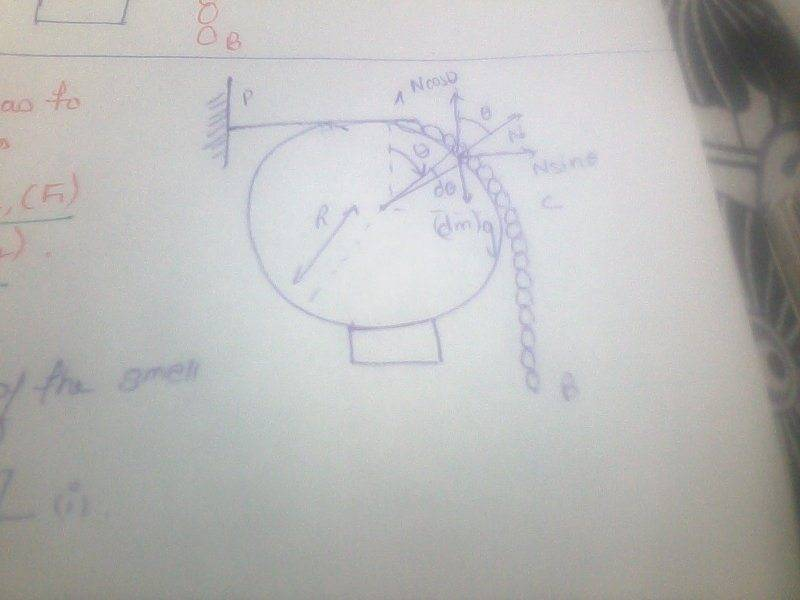# What's wrong with my answer?

## Homework Statement

The question says
A chain of mass per unit length k and length 1.5 m rest on a fixed smooth sphere of radius R= 2/π m

N/a

## The Attempt at a SolutionI think that the tension has to balance two types of forces :
1) rightward force of part AC(touching)
2)downward force of part CB(hanging)
Finding first type of force:
Using Fbd(attached) of small part taken in touching part
Then Ncos(theta)=(dm)g
→ N=(dm)g/cos(th.).......(1)
Length of that part =R(d(th.))
mass of that small part =Rk(d(th.))
Using Fbd rightward force on the small part =Nsin(th.)=(dm)g(tan(th.))
=Rkgtan(th.)(d(th.))
Now if I want to integrate it , i would have to use limits from 0 to π/2
BUT tan (th.) is not integrable b/w 0 to π/2 . So whats wrong with my answer ?

#### Attachments

BvU
Homework Helper
I'm missing something in your force balance for the little chunk of chain. I see an N and an mg and they clearly don't balance. What force could possibly complete the picture ?

I'm missing something in your force balance for the little chunk of chain. I see an N and an mg and they clearly don't balance. What force could possibly complete the picture ?
It could have been friction BUT the sphere is smooth so , what can be the force (you are talking about ) left out that balances the tension of the thread ?

haruspex